# Virtual Arduino Tennis

Play tennis on a Neopixel matrix and an accelerometer with an Arduino! It's like Mario Tennis meets makers!

## Things used in this project

### Hardware componentsArduino Nano R3
×2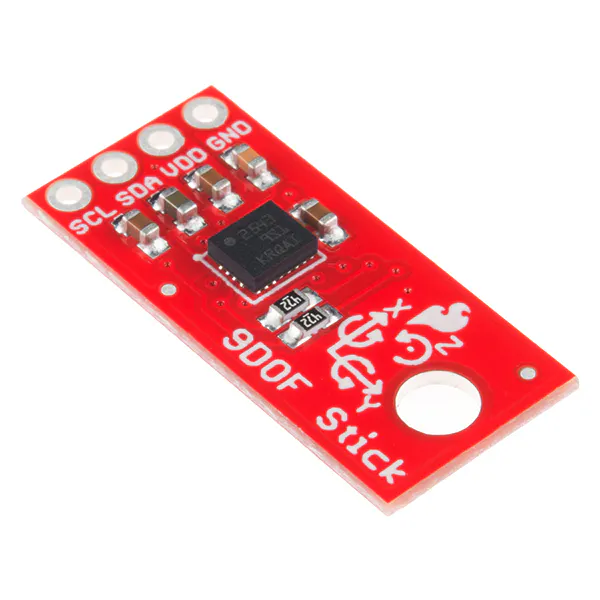SparkFun 9DoF Sensor Stick
×1Jumper wires (generic)
×1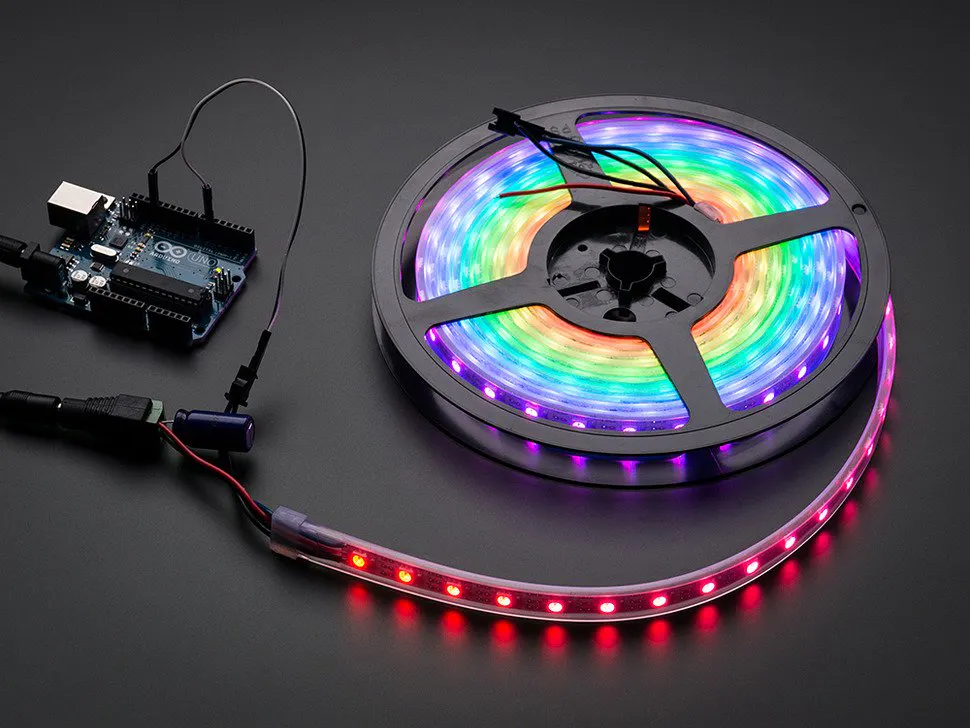NeoPixel strip
×1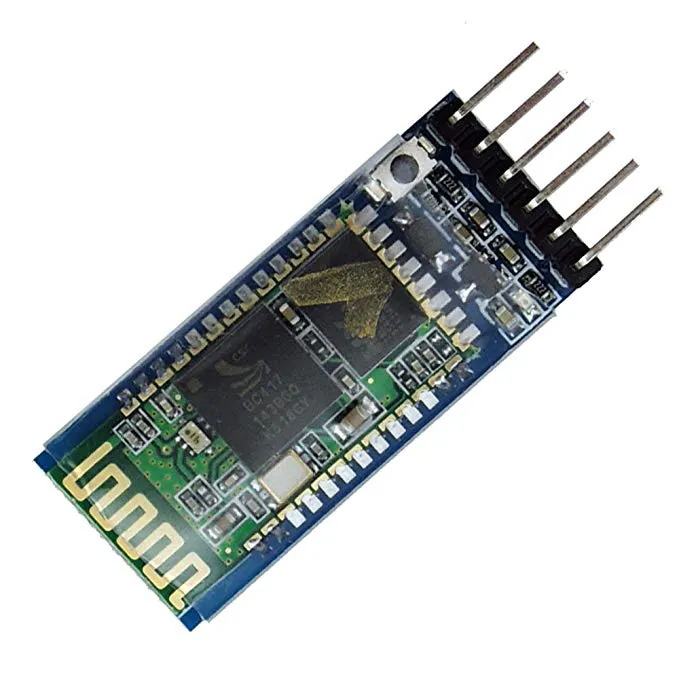HC-05 Bluetooth Module
×1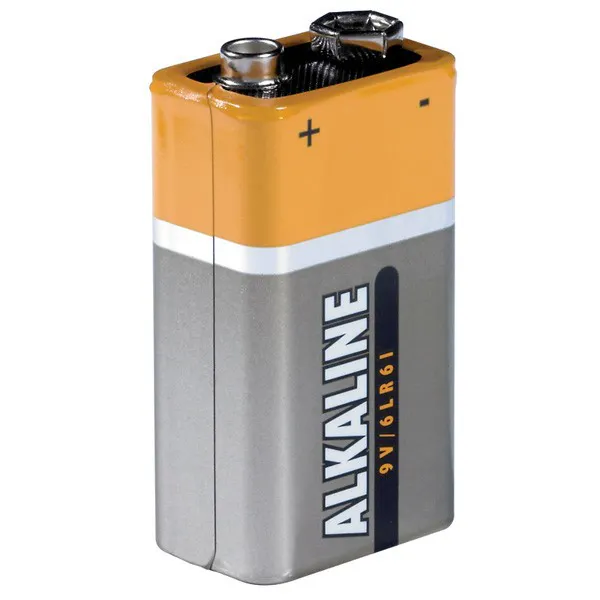9V battery (generic)
×1

### Software apps and online servicesArduino IDE

### Hand tools and fabrication machinesSoldering iron (generic)

## Schematics

### Controller Schematic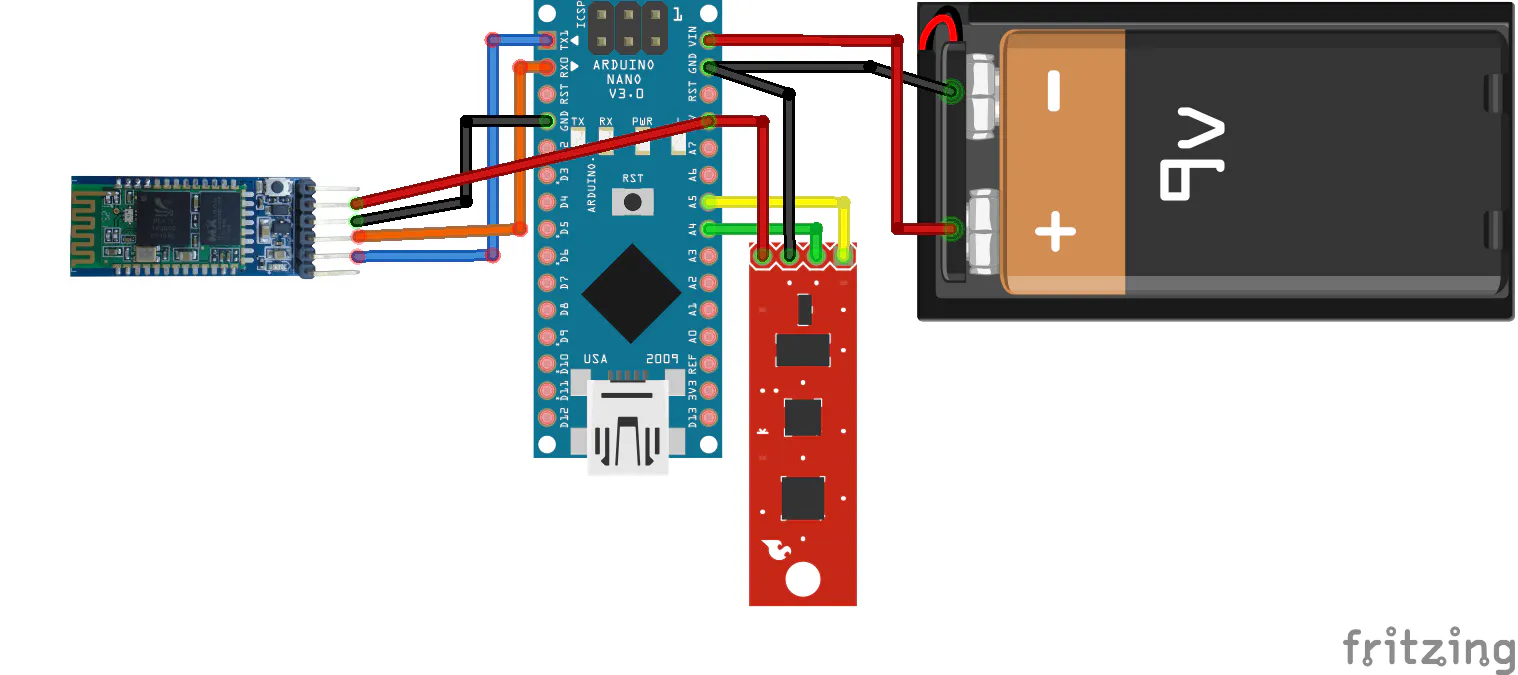### Matrix Schematic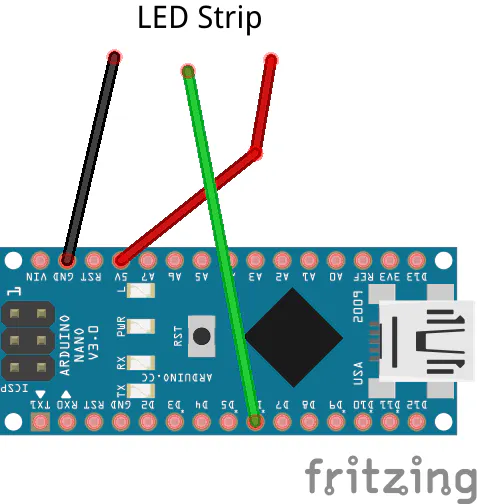## Code

### Matrix Code

C/C++
Goes on the Arduino attached to the matrix.
```#include <Adafruit_GFX.h>
#include <Wire.h>
#include <EEPROM.h>

#define PIN 6 //Data pin for matrix
#define HS_ADR 0x02 //The address of the highscore byte in the EEPROM

#define NOTE_C1  33
#define SPKR_PIN 3

NEO_MATRIX_BOTTOM     + NEO_MATRIX_LEFT +
NEO_MATRIX_COLUMNS + NEO_MATRIX_ZIGZAG,
NEO_GRB            + NEO_KHZ800);

int ball_x = 7; //Ball's X coord
int ball_y = 2; //Ball's Y coord
int r_incr = 1; //How much to increase radius by
bool isSwung = false; //Is the raquet swung
int ball_x_dir = 1; //Ball's X direction
int ball_y_dir = 1; //Ball's Y direction
bool isDirRight = true;
int score = 0; //The score of the current game
int COM_score = 0;
int highscore = 0; //The high score of all games
int framerate = 50; //How many ms between each frame
int serial_data;
String score_string = "";
uint16_t colors[] = {matrix.Color(255,0,0),matrix.Color(0,255,0),matrix.Color(150,200,0)};
int melody[] = {0};
int tempo[] = {0};
static unsigned long lastFrame = 0;

void setup(){
Serial.begin(9600);
matrix.begin();
matrix.fillScreen(0);
matrix.setTextColor(colors);
display_scores();
Serial.println(highscore,DEC);
matrix.setCursor(0,1);
matrix.print("HS: ");
matrix.show();
delay(1000);
matrix.fillScreen(0);
matrix.setCursor(0,1);
matrix.print(highscore,DEC);
matrix.show();
delay(1000);
score_string = "";
}

void loop(){
if((lastFrame+framerate)< millis()){
update_frame();
lastFrame = millis();
}
}

void update_frame(){
serial_data = Serial.parseInt();
if(serial_data){
isSwung = true;
}
else if(!serial_data){
isSwung = false;
}
if(ball_y >= 5 && ball_y < 7 && isSwung){
if(isDirRight){
ball_x_dir = -1;
ball_y_dir = -1;
}
else if(!isDirRight){
ball_x_dir = 1;
ball_y_dir = -1;
}
r_incr = -1;
}
else if(ball_y >= 8){
COM_score += 1;
end_round();

}
else if(ball_y <= 2){
isDirRight = !isDirRight;
int randNum = random(4);
Serial.println(randNum);
if(randNum == 2){ //25% chance of COM missing
score += 1;
if(score > highscore){
write_HS();
}
end_round;
}
else{
if(isDirRight){
ball_x_dir = 1;
ball_y_dir = 1;
}
else if(!isDirRight){
ball_x_dir = -1;
ball_y_dir = 1;
}
r_incr = 1;
}
}
ball_x += ball_x_dir;
ball_y += ball_y_dir;
matrix.fillScreen(0);
matrix.show();
}

void end_round(){

if(COM_score >= 10){
end_game();
}
isDirRight = true;
r_incr = 1;
ball_x_dir = 1;
ball_y_dir = 1;
ball_x = 7;
ball_y = 2;
display_scores();
matrix.fillScreen(0);
matrix.show();

}

void end_game(){
matrix.fillScreen(0);
matrix.setCursor(0,1);
matrix.setTextColor(colors);
matrix.drawLine(3,0,12,9,colors);
matrix.drawLine(11,0,2,9,colors);
matrix.show();
delay(500);
while(1){
}
}

void display_scores(){
matrix.fillScreen(0);
matrix.setTextColor(colors);
matrix.setCursor(0,1);
score_string = String(score) + "-" + String(COM_score);
scrollText(score_string);
matrix.fillScreen(0);
delay(2000);
matrix.show();
}

void scrollText(String text){
int pass = 0;
int x = matrix.width();
for(int i=0;i<24;i++){
matrix.fillScreen(0);
matrix.setCursor(x,2);
matrix.print(text);
x -= 1;
matrix.show();
delay(150);
}
}

void write_HS(){
EEPROM.write(0x04,int(score));
}

return HS;
}
```

### Racquet Code

C/C++
```#include <Wire.h>
#include <SPI.h>
#include <SparkFunLSM9DS1.h>

LSM9DS1 imu;

#define LSM9DS1_M  0x1E // Would be 0x1C if SDO_M is LOW
#define LSM9DS1_AG  0x6B // Would be 0x6A if SDO_AG is LOW

#define PRINT_SPEED 10 // 10 ms between checks
static unsigned long lastPrint = 0; // Keep track of print time

float accelx = 0;
float accely = 0;

void setup() {
// put your setup code here, to run once:
Serial.begin(9600);
imu.settings.device.commInterface = IMU_MODE_I2C;
if (!imu.begin())
{
//failed
while (1)
;
}
}

void loop() {
if ((lastPrint + PRINT_SPEED) < millis())
{
// put your main code here, to run repeatedly:
if ( imu.accelAvailable() )
{
// To read from the accelerometer, first call the
// readAccel() function. When it exits, it'll update the
// ax, ay, and az variables with the most current data.
}
lastPrint = millis(); // Update lastPrint time
}
accelx = imu.calcAccel(imu.ax);
accely = imu.calcAccel(imu.ay);

if(accelx <= -1.5 || accelx >= 1.5){
Serial.print(1);
delay(600);
}
else if(accely <= -1.5 || accely >= 1.5){
Serial.print(1);
delay(600);
}
}
```

### Python Code

Python
```import serial
import time

matrix_port = "COM3"
raquet_port = "COM9"

matrix = serial.Serial(matrix_port, 9600)
racquet = serial.Serial(raquet_port,9600)
time.sleep(10)
while 1:
print data
if data == "1":
print "hit"
matrix.write("1")
time.sleep(1)
time.sleep(.05)
```

## Credits

### Arduino “having11” Guy

82 projects • 539 followers
18 year-old IoT and embedded systems enthusiast. Also an intern at Hackster.io who loves working on projects and sharing knowledge.